﻿ Divergence and Similarity of the Binary Logistic Regression and Linear Discriminant Analysis Models in Evaluating Factors Associated with Bluetongue Virus in CattleInternational Journal of Statistics and Applications

p-ISSN: 2168-5193    e-ISSN: 2168-5215

2019;  9(6): 180-185

doi:10.5923/j.statistics.20190906.02### Divergence and Similarity of the Binary Logistic Regression and Linear Discriminant Analysis Models in Evaluating Factors Associated with Bluetongue Virus in Cattle

Azza B. Musa1, Amal Alsir Alkhidir Abedalraheem2, Mohamed T. Ibrahim2, H. Hamad3, Siddik Mohamed Ahmed Shaheen4

1Central Laboratory, Department of Statistics, Ministry of Higher Education and Scientific Research, Khartoum, Sudan

2Sudan University of Science and Technology, Khartoum, Sudan

3Central Veterinary Research Laboratory, Khartoum, Sudan

4University of Khartoum, College of Economics and Social Studies, Khartoum, Sudan

Correspondence to: Azza B. Musa, Central Laboratory, Department of Statistics, Ministry of Higher Education and Scientific Research, Khartoum, Sudan.
 Email: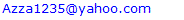Abstract

Binary logistic regression (BLR) and linear discriminant analysis (LDA) are often used to classify populations or groups using a set of predictor variables. The aim of this study is to evaluate the convergence of these two methods when they are applied in non normally distributed epidemiological data. The main criteria used for comparing BLR and LDA were the coefficients of each model, the sample size impact to percentage to correct classification, sensitivity, specificity and accuracy of the models, and the area under the ROC curve (AUC) using ROC curve analysis. Individually, ROC analysis was carried out using the predicted probabilities saved from the two statistical methods. In conclusion, BLR and LDA showed similar results, even with violation of normality assumption.

Keywords: Binary Logistic Regression, BTV, Linear Discriminant Analysis, ROC Curve

Cite this paper: Azza B. Musa, Amal Alsir Alkhidir Abedalraheem, Mohamed T. Ibrahim, H. Hamad, Siddik Mohamed Ahmed Shaheen, Divergence and Similarity of the Binary Logistic Regression and Linear Discriminant Analysis Models in Evaluating Factors Associated with Bluetongue Virus in Cattle, International Journal of Statistics and Applications, Vol. 9 No. 6, 2019, pp. 180-185. doi: 10.5923/j.statistics.20190906.02.

### 1. Introduction

Logistic regression and linear discriminant analysis are multivariate statistical methods which can be used for the evaluation of the associations between various covariates and a categorical outcome. Both methodologies have been extensively applied in research, especially in medical and sociological sciences. Logistic regression is a form of regression which used when the dependent variable is dichotomous, discrete, or categorical, and the explanatory variables are of any kind. In medical sciences, the outcome is usually the presence or absence of a stated situation or a disease .
Choosing the exact statistical method for data fitting is a frequent question for researchers. Among the most paramount criteria for the differentiation between statistical methods are, the type of response variable as well as the purpose of the research design. If we have a categorical and dichotomous dependent variable, both binary logistic regression (BLR) and linear discriminant analysis (LDA) was suggested as the two multivariate models that have been used for classification of cases into their original groups. This multivariate technique can be used to find out which explanatory variable best discriminate between two or more groups along with the classification of cases into their proper group [2,3]. The number of canonical discriminant functions is mainly determined by the number of categories minus one, or the number of discriminators variables, which is smaller. If we have only two groups or categories, then discriminant function will be derived giving the simplest form of LDA. To date, there has been an increasing interest in choosing between BLR and LDA for analysis of biological data. Although the theory behind each method has been extensively published, the comparison between the two approaches still represents a problem for researchers who aimed to distinguish between two or more categorical outcomes in practice . Thus, it can be proposed that both discriminant analysis and logistic regression can be used to predict the probability of a specified outcome using all or a subset of available variables. This study aims to evaluate the convergence and choosing between two methods when they are applied in epidemiological data and set some guidelines for proper choice; this is the problem that motivated this research. The comparison between the methods is based on several measures of predictive accuracy using blue tongue virus data.
Bluetongue virus (BTV) is an infectious disease transmitted by Culicoides biting midges, affecting mainly domestic and wild ruminants, one of the 22 species or serogroups in the genus Orbivirus in the Reoviridae family. BTV causes severe morbidity and mortality in sheep, while the infection is subclinical in some domestic and wild ruminants . BTV is an arbovirus, and until recently, its transmission was thought to be only mediated in cattle and ruminant through the bite of infected midges. This sole transmission route has been challenged recently with the emergence of reports of direct contact transmission with some serotypes and vertical transmission from mother to fetus .
In this study, linear discriminant analysis and binary logistic regression methods are compared using the Blue tongue virus (BTV) data set as a cross sectional study. These data were collected by veterinarian researcher in 2015, from Gedarif state- eastern Sudan, to study the prevalence of virus and risk factors associated with disease in cattle, there are 4 catecorigal predictor variables (Breed, Sex, Locality, Climate), and Age was quantitative variable.

### 2. Material and Methods

Logistic regression is a form of regression which is used when we want to predict probabilities of the presence or absence of a particular disease, characteristic, or an outcome in general based on a set of independent of explanatory variables of any kind (continuous, discrete, or categorical). Since the predicted probability must lie between 0 and 1, simple linear regression techniques are insufficient to achieve that, because they allow the dependent variable to pass these limits and to produce inconsistent results .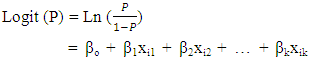(1)
The term p / (1 -p) is the odds ratio [8,9], βj is the value of the jth coefficient, j = 1, 2, 3…, k and xij is the value of the ith case of the jth independent variable. The parameters of BLR are βo, β1 …, βk. By taking the exponential function for the previous equation, the probability of occurrence of a condition can be estimated using the following logistic regression model: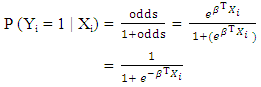(2)
Where Yi is the binary outcome; Xi is the independent variable, the base e is the exponential function, and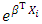is the odds ratio for the independent variable Xi.
Theoretically, BLR is more flexible regarding the assumptions, particularly those of independent variables. However, both methods require some assumptions in common  such as independency of observations, absence of multicollinearity between predictors, and lack of outliers in datasets.
Linear discriminant analysis (LDA) was used to examine the association between a categorical outcome and multiple independent variables in the form of discriminant function. The linear discriminant equation (LDE) given as follows: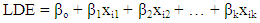(3)
Where βj is the observation of the jth coefficient or weight, j = 1, 2…, k, xij is the observation of the ith animal, for the jth independent variable. Based on estimates of the coefficients of LDA, the explanatory variables are identified to discriminate between the groups of interest. In practice, the coefficients with high magnitude reflect the importance of the corresponding variable in explaining the outcome. Furthermore, this function produces what is called discriminant scores, from which the predicted probabilities will be estimated for each case of the categorical outcome variable. These discriminant scores, along with the group means (centroids) contribute in the classification of cases into their groups .
The data that used in this research was collected by veterinarian researcher for carry out the relationship between dependent variable (BTV result) and independent variables (risk factors).
We compared the classification performance of BLR and LDA, by computing the empirical frequency of correct classified observations over the tested sample, and the methods for comparing the two classifiers was performed statistical comparisons of the accuracies of BLR and LDA classifiers, on the specific datasets.
The resulting models were used for classification, varying the cut-off points or prior probabilities and noting how the sensitivity, specificity and overall classification rate (total correct classification percentage) varies at each cut-off point. After investigating the models, predictive abilities at different cut-off points, the summary statistics were compiled and compared. The comparisons were based on the following aspects: the variables selected in the models, the sign and magnitude of the coefficients, sensitivity, specificity and classification accuracy at varying cut-off probabilities, percentage of correct classification with different sample size. We used standardized and unstandardized function coefficients for discriminant analysis, and Z statistic (squared Wald statistic) for logistic regression , to evaluate two models.
The contribution of respective variables to the discrimination depends on how large the coefficients are, and we also compared the sign and magnitude of coefficients.
The Response Operating Characteristics (ROC) curves were also compared to determine which of the two models enclosed a larger area indicating better classification ability. The ROC curve plots sensitivity (rate of true positives) and 100 minus specificity (rate of true negatives) at several cut-off points and so provides a quick graphical assessment of the effect of varying the cutoff point in any classification model.
All of statistical analysis and computations performed on SPSS software statistical package version 25.0 (SPSS, Inc., Chicago, Ill, USA), and NCSS Data analysis software 2019.

### 3. Results

The first set of analysis in this study was carried out to examine the assumptions required by linear discriminant analysis. Box's M statistic which has been used to test the homogeneity of covariance matrices revealed the violation of that assumption (Box's M = 33.082, F = 2.066, and sig = 0.009) in all analyses. The dataset denoted non-normal distribution for all variables. The results obtained from the preliminary analysis showed no signs of collinearity between the explanatory variables. The highest correlation (0.401) observed between age and sex.
 Table 1. Variables and Coefficients for binary Logistic Regression and the Discriminant Analysis Models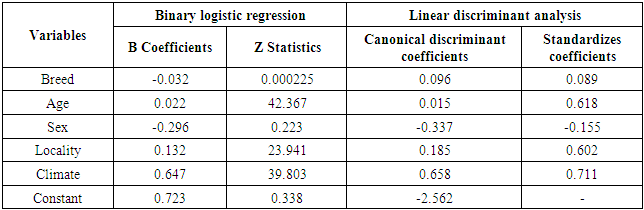From table 2, it can be seen that BLR relied on chi-square distribution and Wald statistic, while LDA used F- distribution and Wilkes lambda statistic for testing the contribution of explanatory variables in discrimination of animals of two groups, in term of determining the best set of predictors which significantly differentiate between positive and negative. Results of both BLR and LDA revealed that age and climate have significant (p < 0.05) contribution in data classification, using the total sample of this study (n = 424) but locality revealed significant contribution in BLR only.
 Table 2. The role of predictors in explaining the outcome using binary logistic regression and linear discriminant analysis models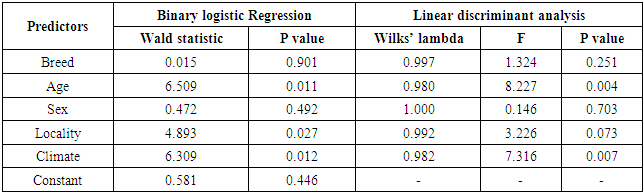After fitting the two models, they were compared on the basis of the variables selected, the sign and magnitude of the coefficients, sensitivity, specificity, overall classification accuracy and the areas enclosed under their respective ROC curves. Thus the cut-off or probability points were varied from 0.1 to 0.9 and the resulting attributes at each point were recorded and illustrated in table (4).
In order to test the effect of sample size on the classification abilities of BLR and LDA, five different random samples were chosen from the studied real dataset. The percentages of correct classification were recorded for the two analytical methods along with the variation in the sample sizes (100, 200, 300, 350, and 424). Referring to the findings in Table 3, the more surprising result to report form this data is that the percent of correct classification of animals were higher when using smaller sample sizes (100), for both BLR and LDA, compared to larger samples (424). The result observed that same ability of BLR and LDA to correctly classify animals for all sample sizes, except the lower sample size (100) the BLR have higher percent (100%) than LDA (98.9%).
 Table 3. Percentages of correct classifications of animals conducted by binary logistic regression and linear discriminant analysis models, at different sample sizes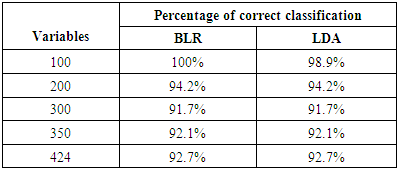Table (4) presents sensitivity, specificity, and accuracy of both approaches at various cutoffs of the probability of having disease. Although, the two models showed typical values of sensitivity, specificity and accuracy at most cutoff points, some differences were observed between the two methods at 0.8 and 0.9 cutoff points (Table 5).
 Table 4. Comparison of binary Logistic Regression and Linear Discriminant Analysis in terms of Sensitivity, Specificity and Classification Accuracy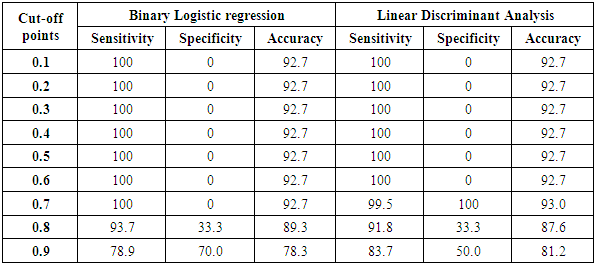Another criterion to compare BLR with LDA was the graphing of Receiver Operating Characteristic (ROC) curve. The accuracy of the test depends on how well the test separates the group being tested into those with and without the disease in question. Accuracy is measured by the area under the ROC curve.
The area under ROC curve (AUC), standard error of (AUC), 95% confidence interval (C.I.) for the area under ROC curve, and the significance test for AUC are presented for each model in Table (5).
 Table 5. Area under the ROC curve (AUC), standard error (SE), 95% confidence interval (CI), and significance tests for linear discriminant analysis and binary logistic regression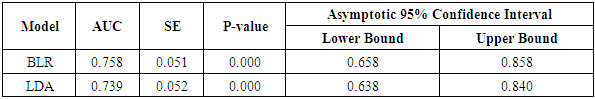In this study, we have plotted the ROC curve for both BLR and LDA, the whole sample (n = 424). As Table 5 shows, the area under ROC curve for BLR was 0.758 (SE = 0.051, 95% C.I = 0.658- 0.858). On the other hand, the area under ROC curve for LDA was 0.739 (SE = 0.052, 95% C.I = 0.638- 0.840).
Figures 1 and 2 presented the ROC curves for BLR and LDA using a real dataset of bluetongue virus with the total examined sample (n = 424). The curves revealed that the differences in AUC for the two models were quite small and may be ignored.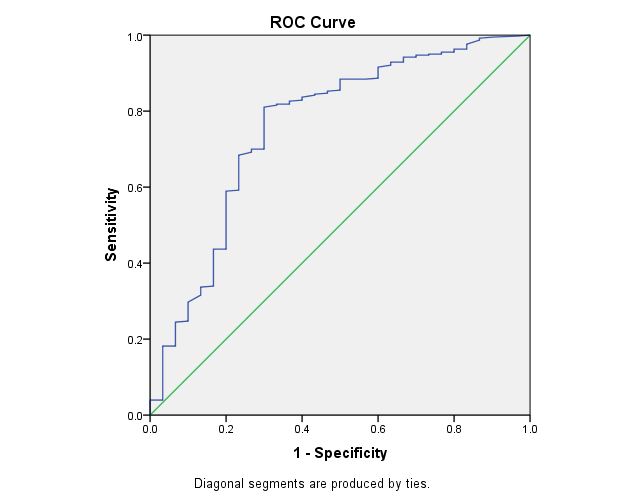Figure 1. ROC curve for binary logistic regression model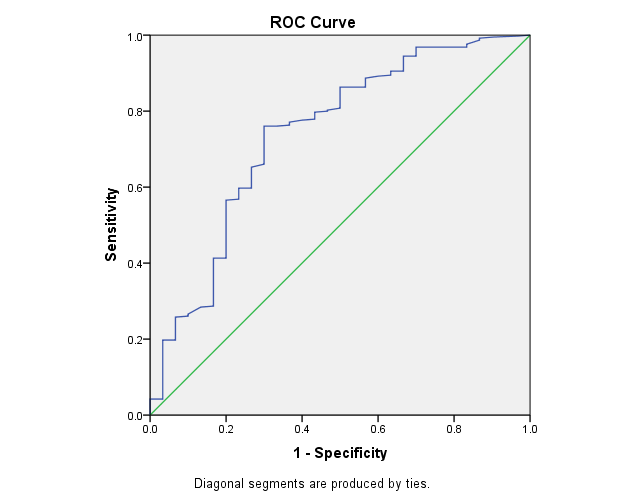Figure 2. ROC curve for linear discriminant model

### 4. Discussion

The present study was designed to evaluate the robustness of linear discriminant analysis and binary logistic regression when categorical data, with special consideration for the outcomes. A real veterinary dataset have been used to compare between the two statistical models. In general, both logistic regression and discriminant analyses converged in similar results. Both methods estimated the same statistical significant coefficients, with similar effect size and direction, Moreover, the results of Wilks' lambda and wald statistics for testing the overall performance of LDA and BLR respectively, confirm the conclusion that both methods are robust when using non-normal data.
Classification of animals having BTV or not was carried out on different sample sizes, results revealed that same percent of correct classification over all sample size, except smallest sample size (100). The results of ROC curve and the area under the curve (AUC) can also be considered as another evidence for evaluating the performance and quality of the LDA and BLR. The findings of ROC curves of this study revealed small difference of AUC between LDA and BLR (decimals).
In term of using real non-normal datasets, there is a similarity between the finding in this study and those described in the literature, Tabachnick B.G. and Fidell L.S & Sueyoshi T. and Hwang [11,12] found that LDA and BLR performed equally in determining the practical differences between groups.
Inconsistent findings have been published about the performance of BLR and LDA with regard to sample size. For example, the study of Wilson and Hargrave reported that LDA was better than BLR when analyzing small size datasets . Moreover, George Antonogeorgos, Demosthenes B. panagiotakos, Kostas N. Priftis and Anastasia Tzonou  concluded that the differences between BLR and LDA may be neglected if we have large sample sizes. They expected that small samples may lead to unstable and invalid estimates. The present findings are in accordance with the results of El-habil A. and El-Jazzar M.A.  who reported that the percent of correct classification was higher in LR than did LDA. They also, indicated that the variation in sample size has the same effect on the two analytical models. The present results seem to be consistent with other research findings. For example, a study was carried out by Zandkarimi E., Safavi, A.A., Rezaei M. and Rajabi G.  for differentiation of normal and diabetic patients using both BLR and LDA. They demonstrated that the classification power was higher for BLR than LDA. Also, Liong and Foo used real datasets to compare BLR and LDA on the basis of normality assumption , number of predictors, and sample size. They mentioned that in general, BLR denoted better results regardless the distribution of explanatory variables. However, they showed that the two methods perform equally with larger samples. On the other hand George Antonogeorgos, Demosthenes B. panagiotakos, Kostas N. Priftis and Anastasia Tzonou [17,1] concluded that both LDA and BLR denoted the same predictive and classification model in the studies that have been conducted on outcomes from health problems. Dealing with veterinary data, one earlier study was performed by Montgomery M.E., White M.E. and Martin S.W.  to evaluate the two methods. The interesting finding of their study was the preference of BLR than LDA especially when the normality assumption and homogeneity of covariance matrices were not verified. The results of ROC curve and the area under the curve (AUC) can also be considered as another evidence for evaluating the performance and quality of the BLR and LDA. Taking sample size into account, it has been recommended that the clinical conclusions from ROC curves can be regarded if the sample size was 100 and more . The findings of ROC curves of this study, AUC revealed that BLR is slightly higher than LDA. Although, the AUC was something larger for BLR than LDA, the significant statistics for testing the AUC for both methods indicate that all AUC were significantly different from half. Therefore, it can be concluded that both LDA and BLR were strongly able to differentiate between cattle infected or not, with regard to the non-normal explanatory variables.
A recent study has been conducted by Ahmadi M.A. and Bahrampour  for examining the differences between LDA and BLR in predicting diabetes using real datasets. Their results showed that AUC for LDA and BLR were similar (0.801 and 0.803, for LDA and BLR, respectively). Similarly, George et al. reported AUC as 0.744 and 0.746, for LDA and BLR, respectively . In general, this study showed that changing the sample sizes lead to nearly similar results, for both LDA and BLR.

### 5. Conclusions

In summary, the first finding that can be drawn from this study was that both methods have selected the same predictors for significant differentiation, using non-normally distributed data. The second major outcome was that the sample size has the same impact on LDA and BLR, regarding the percentages of animals being correctly classified, Although, the area under the roc curve (AUC) showed BLR slight superiority than LDA, and classification accuracy of higher cutoff points also showed small difference between two models.
In conclusion, logistic regression and discriminant analyses were similar in the model analysis. In order to decide which method should be used, we must consider the assumptions for the application of each one.

### ACKNOWLDGEMENTS

I would like to express our gratitude to Central laboratory- Ministry of higher education and scientific research- Khartoum – Sudan for the financial support.

### References

  George Antonogeorgos, Demosthenes B. panagiotakos, Kostas N. Priftis and Anastasia Tzonou, 2009, Logistic Regression and Linear Discriminant Analyses in Evaluating Factors Associated with Asthma Prevalence among 10-12- Years-Old Children: Divergence and Similarity of the Two Statistical Methods, International Journal of Pediatrics, 952042.  Timm, N.H., 2002, Applied multivariate analysis, 2nd ed., Springer Texts in statistics.  Hamid, H., 2010, A new approach for classifying large number of mixed Variables, International Journal of Mathematical, Computational, Physical, Electrical and Computer Engineering 4:10, 1355-1360.  Sherif A. Moawed, Mohamed M. Osman, 2017, The Robustness of Binary Logistic Regression and Linear Discriminant Analysis for the Classification and Differentiation between Dairy Cows and Buffaloes, International Journal of Statistics and Applications 7:6, 304-310.  Jose M Rojas, Daniel Rodríguez-Martín, Verónica Martín and Noemí Sevilla, 2019, Diagnosing bluetongue virus in domestic ruminants: current perspectives, veterinary medicine research and report.  Van der Sluijs MT, de Smit AJ, Moormann RJ., 2016, Vector independent transmission of the vector-borne bluetongue virus, Crit Rev Microbiol 42:1, 57–64.  Pampel F.C., 2000, Logistic Regression: A Primer, Sage. Thousand Oaks, Calif, USA.  Bernard Rosner, 2010, Fundamentals of Biostatistics, Seven edition, Harvard University.  Hosmer D.W and Lemeshow S., 2000, Applied Logistic Regression, 2nd Edition, John Wiley and Sons, Canada.  Worth, A.P. and Cronin, M.T.D., 2003, The use of discriminant analysis, logistic regression and classification tree analysis in the development of classification models for human health effects, Journal of Molecular Structure 622: 97-111.  Tabachnick, B.G. and Fidell, L.S., 1996, Using multivariate statistics, 3rd ed., New York: Harper Collins.  Sueyoshi, T. and Hwang, S.A., 2004, Use of Nonparametric Tests for DEA-Discriminant Analysis: A Methodological Comparison, Asia-Pacific Journal of operational research 21:2, 179-195.  Wilson, R.L. and Hargrave, B.C., 1995, Predicting graduate student success in an MBA program: Regression versus classification, Educational and Psychlogical Measurement 55: 186–195.  El-habil, A. and El-Jazzar, M.A., 2014, Comparative study between linear discriminant analysis and multinomial logistic regression, An-Najah Univ J Res (Humanities) 28:6, 1525-1548.  Zandkarimi, E., Safavi, A.A., Rezaei, M. and Rajabi, G., 2013, Comparison between logistic regression and discriminant analysis in identifying the determinants of type 2 diabetes among prediabetes of Kermanshah rural areas, J Kermanshah Univ Med Sci 17:5, 300-308.  Liong, C.Y. and Foo, S.F., 2013, Comparison of Linear Discriminant Analysis and Logistic Regression for Data Classification, In: Proceedings of the 20th National Symposium on Mathematical Sciences. Putrajaya, Malaysia: AIP Conf Proc, pp. 1159-1165.  Panagiotakos, D.B., 2006, A comparison between Logistic Regression and Linear Discriminant Analysis for the Prediction of Categorical Health Outcomes, International Journal of Statistical Sciences 5: 73-84.  Montgomery, M.E., White, M.E. and Martin, S.W., 1987, A comparison of discriminant analysis and logistic regression for the prediction of Coliform mastitis in dairy cows, Canadian Journal of Veterinary Research 51:4, 495–498.  Metz, C.E., 1978, Basic principles of ROC analysis, Seminars in Nuclear Medicine, 8: 283-298.  Ahmadi, M.A. and Bahrampour, 2015, A Comparison of logistic regression and discriminant analysis in predicting type 2 Diabetes, Iranian Journal of Epidemiology 11:3, 62-69.